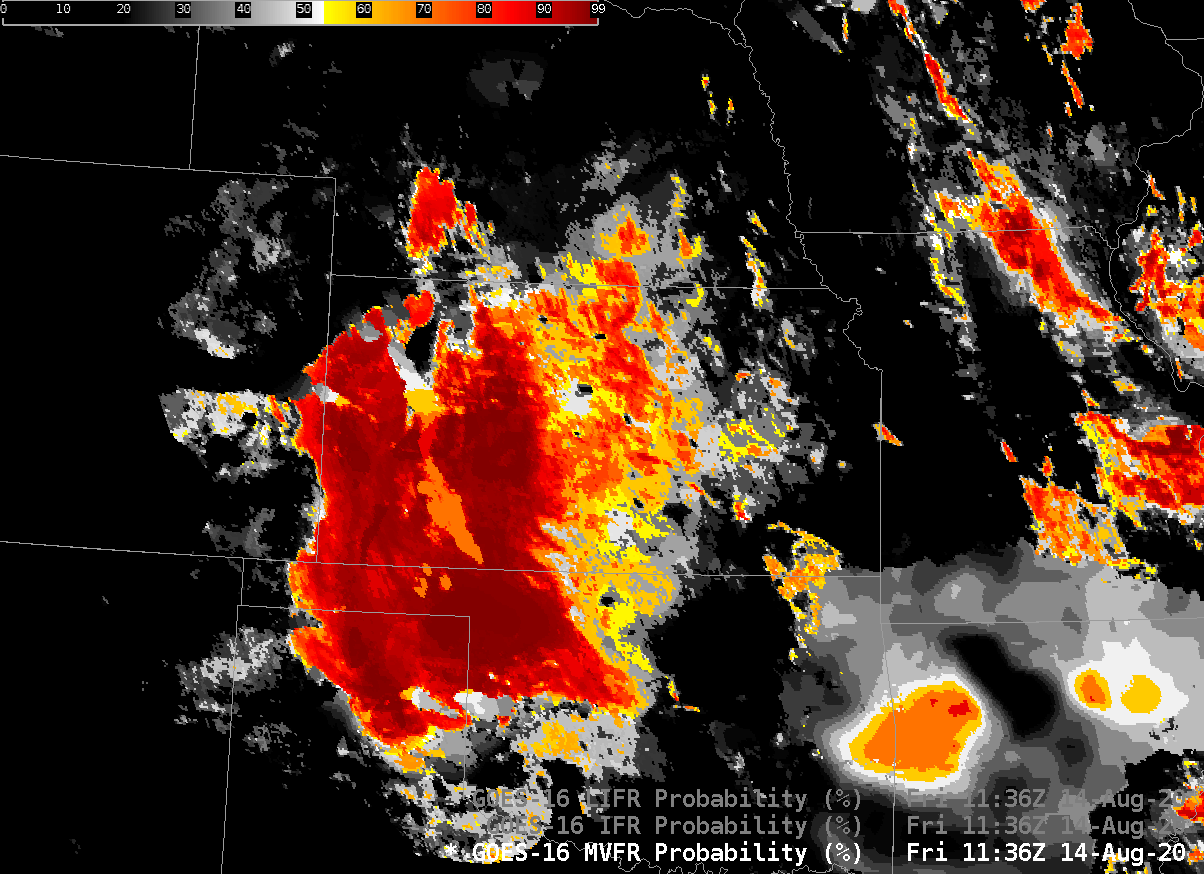# The relationship between MVFR Probability, IFR Probability and Low IFR ProbabilityGOES-16 MVFR Probability, IFR Probability and Low IFR Probability, 1136 UTC on 14 August 2020 (Click to enlarge)

Most of the posts on this blog discuss IFR Probability: The probability that IFR conditions are occurring. IFR, or Instrument Flight Rules conditions are defined as ceilings between 1000 and 3000 feet and/or visibilities between 1 and 3 miles. Two other Probability fields are created: MVFR Probabilities (MVFR, or Marginal Visual Flight Rules, are defined as ceilings between 3000 and 5000 feet and/or visibilities between 3 and 5 statute miles) and Low IFR Probabilities (LIFR, ceilings below 1000 feet and/or visibilities less than 1 mile). The animation above steps through the three fields from one time: MVFR Probability, IFR Probability and Low IFR Probability. As might be expected, MVFR Probability > IFR Probability > LIFR Probability.

Cursor readouts in AWIPS imagery are shown below; LIFR Probability fields are shown with the other two Probability fields are loaded underneath. The cursor readout (for the point just north and west of the upper left corner of the readout values) shows the relationship between the three fields. Low IFR Probability is shown in coral, IFR Probability in green, MVFR Probability in white. MVFR Probability values > IFR Probabilty values > LIFR Probability values.